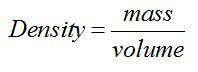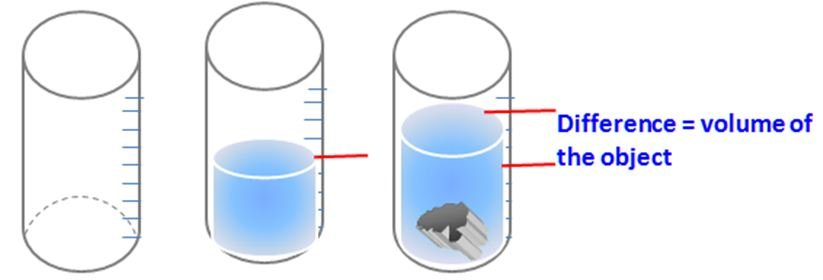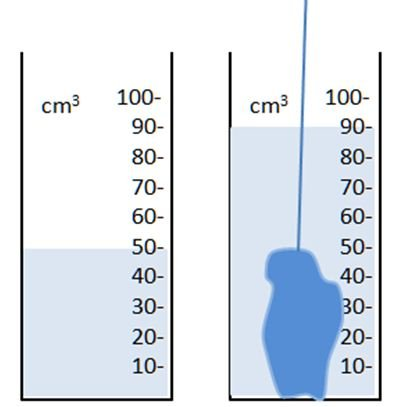# Definition Of Density

## Definition Of Density

"Mass per unit volume of a substance" is known as density.In S.I. system of units mass is measured in Kilograms, and the volume is measured in cubic meters (m3)
The units for density are Kg/ m3
Mass is measured with common balance and the volume is calculated by using different formulae for different types of objects
Example: Volume of a rectangular block = length x breadth x height ( l x b x h).
Find the volume of the following rectangularLength = 4 m : Breadth = 3 m : Height = 1 m ;
Volume of the rectangular block = 4 x 3 x 1 = 12 m3
Volume of Irregular solid (if the shape is not in a regular form) the volume is calculated by using measuring cylinder.

Example: Find the mass of the following objectTake a measuring cylinder.
Fill it partly with water and note the level of water level.
Lower the solid into a partly filled measuring cylinder.
Again note the water level. The rise in level on the volume scale gives the volume of solid. (Final water level � Initial water level).#### Worked Example:

An object of mass 100 g is immersed in water as shown in the diagram
What is the density of the material from which the object is made?A. 0.4g/cm3
B. 0.9g/cm3
C. 1.1g/cm3
D. 2.5g/cm3